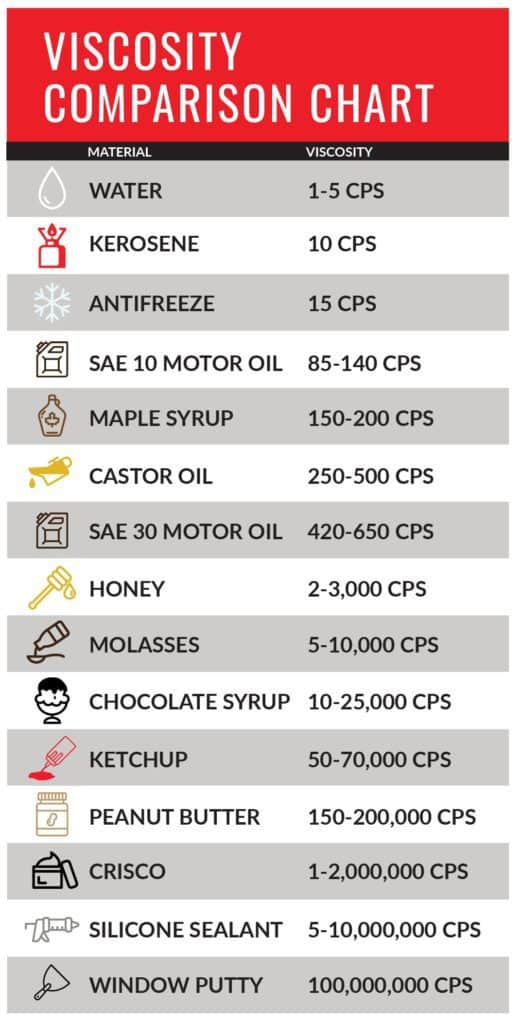## Let it Flow: How to Calculate Viscosity0
access_time

Viscosity is the measure of a material’s resistance to motion under an applied force. There are several formulas and equations to calculate viscosity, the most common of which is Viscosity = (2 x (ball density – liquid density) x g x a^2) ÷ (9 x v), where g = acceleration due to gravity = 9.8 m/s^2, a = radius of ball bearing, and v = velocity of ball bearing through liquid.

If you want determine viscosity in a liquid, try a simple science experiment by measuring the speed of a metal ball dropped in a container of liquid. The velocity of the ball, combined with the relative densities of the ball and the liquid, can be used to calculate the viscosity of liquids. But you can’t calculate viscosity unless you know what it is and why it’s important.

## Definition of Viscosity

The definition of viscosity is “the quantity that describes a fluid’s resistance to flow. Fluids resist the relative motion of immersed objects through them as well as to the motion of layers with differing velocities within them.” Knowing how thick your material is can have huge impact on your ability to get work done. Material such as honey or glue tend to be thicker and are described as having a higher viscosity, whereas water and glycol are less viscous and flow faster.

Temperature also plays a critical role in the viscousness of a liquid. Generally speaking, the warmer a liquid is, the lower its  viscosity is and the easier it flows. The colder it is, the more sludge-like it becomes and has a higher viscosity. Understanding how to calculate viscosity can be helpful when deciding if your material is ready to use or not. So how do you determine the viscosity?## Try This Out

### Calculating the Density of the Ball

1. Measure the mass of your ball, using your balance. For instance, suppose the mass of the ball is 0.1 kilograms (kg).
2. Find the radius of the ball by first measuring the diameter (distance of a straight line through the ball at the widest part). Divide the diameter by 2; this gives the radius of your ball.
3. Calculate the volume of the ball by plugging the radius into the equation for the volume of a sphere. Suppose the ball bearing has a radius of 0.01 meter (m). The volume would be:  Volume = 4/3 x pi x (0.01 m) ^3 = 0.00000419 m^3
4. Calculate the density of the ball by dividing its mass by its volume. The density of the ball in the example would be:  Density = 0.1 kg ÷ 0.00000419 m^3 = 23,866 kg/m^3

### Calculating the Density of the Liquid

1. Measure the mass of your graduated cylinder when it is empty. Then measure the mass of your graduated cylinder with 100 millilters (mL) of liquid in it. Suppose the empty cylinder had a mass of 0.2 kg, and with fluid its mass was 0.45 kg.
2. Determine the mass of the fluid by subtracting the mass of the empty cylinder from the mass of the cylinder with the fluid. In the example:  Mass of liquid = 0.45 kg – 0.2 kg = 0.25 kg
3. Determine the density of the fluid by dividing its mass by its volume. Example:  Density of fluid = 0.25 kg ÷ 100 mL = 0.0025 kg/mL = 0.0025 kg/cm^3 = 2,500 kg/m^3*
4. 1 mL is equal to 1 cm^3 *1 million cubic centimeters equal 1 cubic meter

###Calculate Viscosity of Liquid

1. Fill your tall graduated cylinder with the liquid to be tested so it is about 2 cm from the top of the cylinder. Use your marker to make a mark 2 cm below the surface of the liquid. Mark another line 2 cm from the bottom of the cylinder.
2. Measure the distance between the two marks on the graduated cylinder. Suppose that the distance is 0.3 m.
3. Let the ball go on the surface of the liquid and use your stopwatch to time how long it takes for the ball to fall from the first mark to the second mark. Suppose it took the ball 6 seconds to fall the distance.
4. Calculate the velocity of the falling ball by dividing the distance it fell by the time it took. In the example:  Velocity = 0.3 m ÷ 6 s = 0.05 m/s

Calculate viscosity of liquid from the data you have collected:

1. Viscosity = (2 x (ball density – liquid density) x g x a^2) ÷ (9 x v), where g = acceleration due to gravity = 9.8 m/s^2, a = radius of ball bearing, and v = velocity of ball bearing through liquid.
2. Plug your measurements into the equation to calculate the viscosity of the liquid. For the example, the calculation would look like this:  Viscosity = (2 x (23,866 – 2,500) x 9.8 x 0.01^2) ÷ (9 x 0.05) = 93.1 pascal seconds.

### Viscosity Calculation Formula:

viscosity = shear stress / shear rate

The result is typically expressed in centipoise (cP), which is the equivalent of 1 mPa s (millipascal second).

## Powerblanket Solutions

Powerblanket makes it easy to lower the viscosity of many industrial fluids by heating the container evenly to the desired temperature, allowing your material to achieve the preferred viscousness for use. This saves you time and money, getting back to work instead of waiting for external temperatures to naturally warm up your material.

Powerblanket offers various ready-to-ship viscosity products, from bucket and drum heaters to IBC tote heaters. We can also produce custom solutions for most applications. If you need better flowing fluids, Powerblanket has you covered.